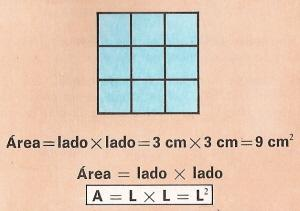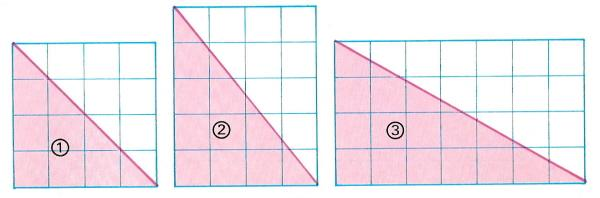Area of parallelogram and triangles1. Area of Rectangle
The upper rectangle is 5 cm width and 3 cm height. How many cm2 is it? Count the squares of a cm2. We obtain 15 cm2.
Area = 5 cm x 3 cm = 15 cm2. Area = width x height; A = b x a.
The area of the rectangle is given by multiplying the width times the height.
The height of the rectangle is equal to the area divided by the width.
The width of the rectangle is equal to the area divided by the height.

Solve these problems on a paper and answer in cm2 or cm:

 The width of a rectangle is 7 cm and its height is 3 cm. What is its area? The area of a rectangle is 102 cm2 and its width is 51 cm. How long is its height? The area is 660 cm2 and its height is 20 cm. How long is its width? A rectangle is 345 cm2 and its width is 23 cm. How long is its height? The width of a rectangle is 7 cm and its height is 6 cm. What is its area?2. Area of square
The square has four equal sides and four right angles. The side of the square is 3 cm. the area will be 3 cm x 3 cm = 9 cm2.
The area of a square is equal to its side-length multiplied by its side-length.
The perimeter of a polygon is equal to the sum of the length of all sides.

Answer these problems in m or m2:

 The side of a square is 5 m. What is its area? What is the perimeter of the previous square? A square-shaped courtyard is 60 m side. What is its area? What is the perimeter of the previous square? A square classroom of a school is 8 m side. What is its area? What is the perimeter of the previous classroom?3. Area of triangle
The square 1 is 4 cm side, so its area will be 16 cm2. When we draw a diagonal the resulting triangle is 8 cm2. You can count them. The area of the square is twice the triangle. So the area of the triangle is base x height divided by 2.

Solve these problems on a paper and answer in cm2:

 The base of a triangle is 14 cm and its height is 8 cm. What is its area? The legs of a right triangle are 9 cm and 7 cm. Find the area. The base of an obtuse triangle is 12 cm and its height is 7 cm. How long is its area?4. Area of a rhomboid
The rhomboid is a parallelogram that has not right angles. In the picture, we cut the scratched left part and we stick it on the right side. We obtain a rectangle with the same base (5 cm) and the same height (3 cm).
The area of the rhomboid is equal to its base multiplied by its height.
Answers these problems in cm2.
 The base of a rhomboid is 7 cm and its height is 4 cm. What is its area? How long is a rhomboid of 12 cm base and 5 cm height? The base of a rhomboid is 8 cm and its height is 4 cm. How long is its area?5. Exercise: answer in cm2 or cm:

 What is the area of the rectangle A? What is the perimeter of the figure A? Find the area of the triangle B. The perimeter of the triangle B is... The area of the square C is... The perimeter of C is... Find the area of the rectangle D Find the perimeter of D The area of the square E is... The perimeter of the square E is... Find the area of the triangle F The perimeter of F is...

 .adslot_S1 { display:inline-block; width: 336px; height: 280px; } @media (max-device-width:970px) { .adslot_S1 { display: none; } } If you are registered, please enter your data:   Name:  Password:   | Educational applications | Mathematics | In Spanish  | To print

«Arturo Ramo GarcĒa.-Record of intellectual property of Teruel (Spain) No 141, of 29-IX-1999
Plaza Playa de Aro, 3, 1║ DO 44002-TERUEL